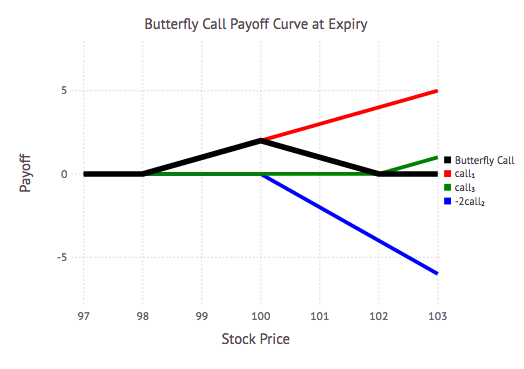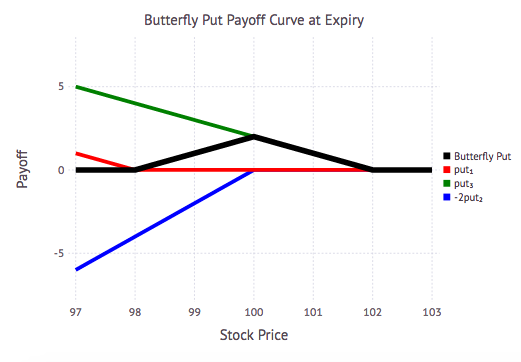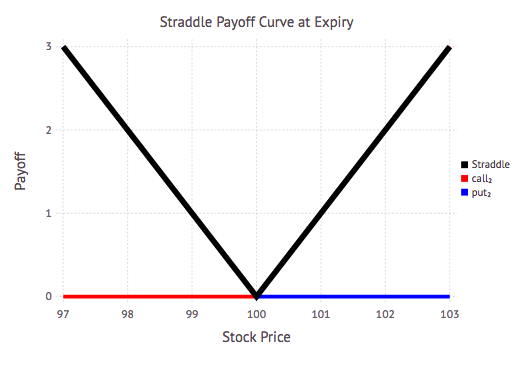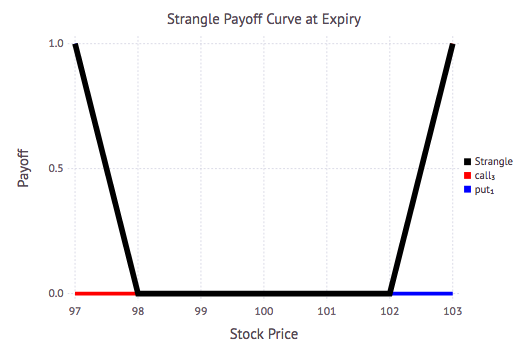Miletus is a financial contract definition, modeling language, and valuation framework written in Julia. Such contract definition languages originated in research papers by Peyton Jones and Eber [PJ&E2000], [PJ&E2003].

Miletus allows for complex financial contracts to be constructed with a combination of simple primitive components and operations. When viewed through the lens of functional programming, these primitive objects and operations form a set of “combinators” that can be used to construct more complex financial constructs.

Miletus provides both primitives for defining financial contract payoffs as well as a decoupled set of valuation model routines that can be applied to combinations of contract primitives. In Miletus, these “combinators” are implemented using Julia’s user-defined types, generic programming, and multiple dispatch capabilities.

Some existing implementations of financial contract modeling environments (created in languages such as Haskell or OCaml) rely heavily on pure functional programming for the contract definition language, but may then switch to a second language (e.g. C++, Java, APL) for implementation of valuation processes, making the implementations opaque. Miletus leverages Julia’s strong type system and multiple dispatch capabilities to both express these contract primitive constructs and generate efficient valuation code. As seen in other parts of the Julia ecosystem, Miletus solves the two language problem with regards to defining and modeling financial contracts.

Example Contract Definition and Valuation

Below we define and value a contract for a European call option, defined both in terms of a set of primitive contract types, as well as convenient, high-level constructors.

``````# Import the library
In: using Miletus
``````

Now we can create a few basic operations using the type constructors provided by Miletus.

``````# Receive an amount of 100 USD
Out: Amount
└─100USD

# Pay is the opposite of Receive
In: x=Pay(100USD)
Out: Give
└─Amount
└─100USD

# Models are constructed and valued on a generic SingleStock type
In: s=SingleStock()
Out: SingleStock
``````

These basic primitives can be combined into higher level operations.

``````# Acquisition of a stock by paying 100USD
In: x=Both(s, Pay(100USD))
Out: Both
├─SingleStock
└─Give
└─Amount
└─100USD

# Which is equivalent to buying the stock
Out: Both
├─SingleStock
└─Give
└─Amount
└─100USD

# The notion of optionality is expressed by a choice of two outcomes
In: x=Either(s, Zero())
Out: Either
├─SingleStock
└─Zero
``````

Another important aspect of any contract is the notion of time. Below we define a temporal condition on which to receive a payment of 100USD.

`````` In: x=When(At(Date("2017-12-25")), Receive(100USD))
Out: When
├─{==}
│  ├─DateObs
│  └─2017-12-25
└─Amount
└─100USD
``````

Combining that temporal condition with optionality defines a basic European Call option.

`````` In: x=When(At(Date("2017-12-25")), Either(Buy(s, 100USD), Zero()))
Out: When
├─{==}
│  ├─DateObs
│  └─2017-12-25
└─Either
├─Both
│  ├─SingleStock
│  └─Give
│     └─Amount
│       └─100USD
└─Zero

In: eucall = EuropeanCall(Date("2017-12-25"), SingleStock(), 100USD)
Out: When
├─{==}
│  ├─DateObs
│  └─2017-12-25
└─Either
├─Both
│  ├─SingleStock
│  └─Give
│     └─Amount
│       └─100USD
└─Zero
``````

Pricing an option requires the use of a valuation model. Below we define a simple Geometric Brownian Motion model, and execute the valuation of our European call option using that model.

`````` In: gbmm = GeomBMModel(today(), 100.0USD, 0.1, 0.05, .15)
Out: Geometric Brownian Motion Model
-------------------------------
S₀ = 100.0USD
T = 2017-03-14
Yield Constant Continuous Curve with r = 0.1, T = 2017-03-14
Carry Constant Continuous Curve with r = 0.05, T = 2017-03-14
σ = 0.15

In: value(gbmm, eucall)
Out: 7.054679704161716USD
``````

Having defined a basic call option, more complex payoff strategies can be created through combinations of multiple options. Spread options consist of combinations of call and put options having different strike prices and/or expiry dates. Below are examples of a few different common spread option strategies.

First, let’s load the packages we will need to construct, visualize, and value our spreads.

`````` In: using Miletus, Gadfly, Colors
import Miletus: Both, Give, Contract, WhenAt, value
``````

Next, let’s define a few parameters that we will use when constructing the different component options of our spread payoffs, and valuation model, including a range of strike prices we will use to visualize the payoff curves.

`````` In: expirydate = Date("2017-12-25")
startdate  = Date("2017-12-1")
interestrate = 0.05
carryrate    = 0.1
Volatility   = 0.15
K₁ = 98.0USD
K₂ = 100.0USD
K₃ = 102.0USD
L  = 11 # Layers in the binomial lattice / Number of time steps
price = K₁-1USD:0.1USD:K₃+1USD
``````

Then we define a function that calculates the payoff curve over a range of prices at a given expiry date:

`````` In: function payoff_curve(c, d::Date, prices)
payoff = [value(GeomBMModel(d, x, 0.0, 0.0, 0.0), c) for x in prices]
p = [x.val for x in payoff]
r = [x.val for x in prices]
return r, p
end
``````

A butterfly call spread consists of four options whose combination pays off in a range of strike prices surrounding a central strike price. It can be built by buying two call options at the high and low strike price and selling two call options at the central strike price.

`````` In: function butterfly_call(expiry::Date, K₁, K₂, K₃)
@assert K₁ < K₂ < K₃
c₁ = EuropeanCall(expiry, SingleStock(), K₁)
c₂ = EuropeanCall(expiry, SingleStock(), K₂)
c₃ = EuropeanCall(expiry, SingleStock(), K₃)
Both(Both(c₁,c₃), Give(Both(c₂,c₂)))
End

In: bfly₁ = butterfly_call(expirydate, K₁, K₂, K₃)

In: s,p_bfly₁ = payoff_curve(bfly₁, expirydate, price)
blk = colorant"black"
red = colorant"red"
grn = colorant"green"
blu = colorant"blue"
plot(layer( x=s ,y=p_bfly₁,Geom.line,Theme(default_color=blk,line_width=1.5mm)),
layer( x=s₁,y=  cp₁  ,Geom.line,Theme(default_color=red,line_width=1.0mm)),
layer( x=s₃,y=  cp₃  ,Geom.line,Theme(default_color=grn,line_width=1.0mm)),
layer( x=s₂,y=-2cp₂  ,Geom.line,Theme(default_color=blu,line_width=1.0mm)),
Guide.manual_color_key("",["Butterfly Call", "call₁", "call₃", "-2call₂"],
["black", "red", "green", "blue"]),
Guide.title("Butterfly Call Payoff Curve at Expiry"),
Guide.xlabel("Stock Price"), Guide.ylabel("Payoff"))
``````The plot above shows payoff curves for both the butterfly call spread (in black) and its constituent call and put options.

A similar combination of put options can be used to construct the same payoff spread as a butterfly put option.

`````` In: function butterfly_put(expiry::Date, K₁, K₂, K₃)
@assert K₁ < K₂ < K₃
p₁ = EuropeanPut(expiry, SingleStock(), K₁)
p₂ = EuropeanPut(expiry, SingleStock(), K₂)
p₃ = EuropeanPut(expiry, SingleStock(), K₃)
Both(Both(p₁,p₃), Give(Both(p₂,p₂)))
end

In: bfly₂ = butterfly_put(expirydate, K₁, K₂, K₃)
s,p_bfly₂ = payoff_curve(bfly₂, expirydate, price)
blk = colorant"black"
red = colorant"red"
grn = colorant"green"
blu = colorant"blue"
plot(layer( x=s ,y=p_bfly₂,Geom.line,Theme(default_color=blk,line_width=1.5mm)),
layer( x=s₁,y=  pp₁  ,Geom.line,Theme(default_color=red,line_width=1.0mm)),
layer( x=s₃,y=  pp₃  ,Geom.line,Theme(default_color=grn,line_width=1.0mm)),
layer( x=s₂,y=-2pp₂  ,Geom.line,Theme(default_color=blu,line_width=1.0mm)),
Guide.manual_color_key("",["Butterfly Put", "put₁", "put₃", "-2put₂"],
["black", "red", "green", "blue"]),
Guide.title("Butterfly Put Payoff Curve at Expiry"),
Guide.xlabel("Stock Price"), Guide.ylabel("Payoff"))
``````Unlike a butterfly spread, straddles and strangles are options spreads with a non-zero payoff outside of a central band of strike prices.

A straddle can be constructed by purchasing both a put and a call having the same strike price and expiry date:

`````` In: function straddle(expiry::Date, K)
p = EuropeanPut(expiry, SingleStock(), K)
c = EuropeanCall(expiry, SingleStock(), K)
Both(p, c)
end

r,p_strd₁ = payoff_curve(strd₁, expirydate, price)
blk = colorant"black"
red = colorant"red"
blu = colorant"blue"
plot(layer( x=s ,y=p_strd₁,Geom.line,Theme(default_color=blk,line_width=1.5mm)),
layer( x=s₁,y=cp₂    ,Geom.line,Theme(default_color=red,line_width=1.0mm)),
layer( x=s₂,y=pp₂    ,Geom.line,Theme(default_color=blu,line_width=1.0mm)),
["black", "red", "blue"]),
Guide.xlabel("Stock Price"), Guide.ylabel("Payoff"))
``````Similarly, a strangle spread can be constructed by purchasing a put option at a low strike price and a call option at a higher strike price.

``````  In: function strangle(expiry::Date, K₁, K₂)
p = EuropeanPut(expiry, SingleStock(), K₁)
c = EuropeanCall(expiry, SingleStock(), K₂)
Both(p, c)
end

In: strangle₁ = strangle(expirydate, K₁, K₃)
r,p_strangle = payoff_curve(strangle₁, expirydate, price)
blk = colorant"black"
red = colorant"red"
blu = colorant"blue"
plot(layer( x=s ,y=p_strangle,Geom.line,Theme(default_color=blk,line_width=1.5mm)),
layer( x=s₁,y=cp₃       ,Geom.line,Theme(default_color=red,line_width=1.0mm)),
layer( x=s₂,y=pp₁       ,Geom.line,Theme(default_color=blu,line_width=1.0mm)),
Guide.manual_color_key("",["Strangle", "call₃", "put₁"],
["black", "red", "blue"]),
Guide.title("Strangle Payoff Curve at Expiry"),
Guide.xlabel("Stock Price"), Guide.ylabel("Payoff"))
``````Each of these spread options can be valued using a single valuation model:

`````` In: gbmm = GeomBMModel(startdate, K₂, interestrate, carryrate, volatility)
Out: Geometric Brownian Motion Model
-------------------------------
S₀ = 100.0USD
T = 2017-12-1
Yield Constant Continuous Curve with r = 0.1, T = 2017-12-1
Carry Constant Continuous Curve with r = 0.05, T = 2017-12-1
σ = 0.15

In: value(gbmm, bfly₁)
Out: 0.40245573232657295USD

In: value(gbmm, bfly₂)
Out: 0.40245573232657295USD

In: value(gbmm, strd₁)
Out: 3.064820288046067USD

In: value(gbmm, strangle₁)
Out: 1.473840565891766USD
``````

Calculating Greeks via Automatic Differentiation

Automatic differentiation (also known as algorithmic differentiation or simply “AD”) is a powerful method for computing gradients and higher order derivatives of numerical programs, which are both numerically exact, yet incur very little computational overhead. A full review article on the use of Automatic Differentiation in Julia to calculate Greeks will be available in an upcoming edition of Wilmott Magazine.

Below we provide a short example of how use of Automatic Differentiation is integrated when calculating the delta of a contract at the start date of the model. Here is the function definition for delta, as included in Miletus:

`````` In: function delta(m::GeomBMModel, c::Contract; autodiff = true)
s = m.core.startprice
s = Dual(s, 1)
vol = m.volatility
gbmm = GeomBMModel(m.core.carrycurve.reference_date, s,
m.core.yieldcurve.rate, m.core.carrycurve.rate, vol)
val = value(gbmm, c)
deriv = extract_derivative(val)
currval(deriv)
end
``````

In this definition, we see that the `startprice` has been extracted from the Geometric Brownian Motion model and converted into a number of type `Dual`. A new Geometric Brownian Motion Model is subsequently created using the parameters of the existing model, but using this `Dual` number for the start price. When the contract is subsequently priced using the `value` function, the inclusion of the `Dual` number in the Geometric Brownian Motion model triggers the calculation of both the current price of the option at each node in the binomial lattice, as well as the calculation of the exact first derivative of the option with respect to stock price at each node. The value function returns both the option price and the option’s delta at the start date. The `delta` value is subsequently extracted and its value is returned from the function.

Use of automatic differentiation in the calculation of greeks allows for determining the exact value of various “derivatives” (in the calculus sense) with a minimal amount of computational overhead, and without being subject to either discretization errors or subtractive cancellation errors present with finite difference approximations.

Performance Comparisons

QuantLib is a well established open source C++ library with functionality for many areas of derivatives pricing and quantitative finance. Below we include an implementation of a performance benchmark comparing the execution of a Cox-Ross-Rubenstein model for pricing an American put option using both Miletus and QuantLib.

First, we show how one can go about defining a function to call QuantLib from a C application:

``````#include <ql/quantlib.hpp>
using namespace QuantLib;

extern "C" {

double ql_am_put_crr(double S, double K, double r, double c, double sigma,
int y1, int m1, int d1, int y2, int m2, int d2, int n) {

Calendar calendar = TARGET();
Date todaysDate(d1, Month(m1), y1);
Date settlementDate(d1, Month(m1), y1);
Settings::instance().evaluationDate() = todaysDate;

Option::Type type(Option::Put);
Date maturity(d2, Month(m2), y2);
DayCounter dayCounter = Actual365Fixed();

Handle<Quote> underlyingH(
boost::shared_ptr<Quote>(new SimpleQuote(S)));
Handle<YieldTermStructure> flatTermStructure(
boost::shared_ptr<YieldTermStructure>(
new FlatForward(settlementDate, r, dayCounter)));
Handle<YieldTermStructure> flatDividendTS(
boost::shared_ptr<YieldTermStructure>(
new FlatForward(settlementDate, c, dayCounter)));
Handle<BlackVolTermStructure> flatVolTS(
boost::shared_ptr<BlackVolTermStructure>(
new BlackConstantVol(settlementDate, calendar, sigma, dayCounter)));
boost::shared_ptr<StrikedTypePayoff> payoff(
new PlainVanillaPayoff(type, K));

boost::shared_ptr<Exercise> americanExercise(
new AmericanExercise(settlementDate, maturity));
boost::shared_ptr<BlackScholesMertonProcess> bsmProcess(
new BlackScholesMertonProcess(underlyingH, flatDividendTS,
flatTermStructure, flatVolTS));

VanillaOption americanOption(payoff, americanExercise);

americanOption.setPricingEngine(boost::shared_ptr<PricingEngine>(
new BinomialVanillaEngine<CoxRossRubinstein>(bsmProcess, n)));

return americanOption.NPV();
}
}
``````

Next, we see both how to call that C function from Julia using `ccall`, as well as an implementation of the CRR model for an American put option implement in Julia, outside of the Miletus framework.

``````# benchmark.jl

import Base.Dates: Date, year, month, day
cd(dirname(@__FILE__))

function ql_am_put_crr(S,K,r,c,σ,dt1::Date,dt2::Date, n)
ccall((:ql_am_put_crr, :quantlib), Cdouble,
(Cdouble, Cdouble, Cdouble, Cdouble, Cdouble,
Cint, Cint, Cint,
Cint, Cint, Cint,
Cint),
S, K, r, c, σ,
year(dt1), month(dt1), day(dt1),
year(dt2), month(dt2), day(dt2),
n)
end

function jl_am_put_crr(S,K,r,c,σ,dt1,dt2,N)
t = Int(dt2 - dt1)/365
Δt = t/N
u = exp(σ*√Δt)
d = exp(-σ*√Δt)
B = exp((r-c)*Δt)
p = (B-d)/(u-d)
q = (u-B)/(u-d)
iR = exp(-Δt*r)

# final time
Z = map(0:N) do i
x = K - S*exp((2*i-N)σ*√Δt)
max(x,0)
end

for n = N-1:-1:0
for i = 0:n
x = K - S*exp((2*i-n)σ*√Δt)
y = iR*(q*Z[i+1] + p*Z[i+2])
Z[i+1] = max(x, y)
end
end
return Z
end
``````

Finally, we can execute the Miletus, QuantLib and plain Julia versions of these functions using functionality from the BenchmarkTools package. BenchmarkTools provides a framework for writing and running groups of benchmarks as well as comparing benchmark results.

``````# benchmark.jl (continued)

using BenchmarkTools
using Miletus

println("CRR Model")
println("=========")
println()
println("Miletus")
println(@benchmark value(CRRModel(Date(2010,01,01), Date(2011,01,01), 801, 36.0, 0.06, 0.0, 0.2),
AmericanPut(Date(2011,01,01), SingleStock(), 40.0)))

println("Miletus Currency")
println(@benchmark value(CRRModel(Date(2010,01,01), Date(2011,01,01), 801, 36.0USD, 0.06, 0.0, 0.2),
AmericanPut(Date(2011,01,01), SingleStock(), 40.0USD)))

if isfile("quantlib")
println()
println("QuantLib")
println(@benchmark ql_am_put_crr(40.0, 36.0, 0.06, 0.0, 0.2,
Date(2010,01,01), Date(2011,01,01), 801))
end

println()
println("Plain Julia")
println(@benchmark jl_am_put_crr(40.0, 36.0, 0.06, 0.0, 0.2,
Date(2010,01,01), Date(2011,01,01), 801))
``````

Execution of our benchmark script yields the following results:

``````julia> include("benchmark.jl")

## CRR Model

Miletus
Trial(10.632 ms)
Miletus Currency
Trial(4.691 ms)

QuantLib
Trial(15.724 ms)

Plain Julia
Trial(4.955 ms)
``````

Summary

Miletus provides functionality for teams across an organization, both front office and back office, to use a common language for structuring, valuing and managing complex financial instruments. Whether a sales team structuring new products, an operations team deploying infrastructure for batch processing, a risk management team determining exposure of a firm’s portfolio, or regulators evaluating capital requirements for solvency, Miletus allows for everyone to use the same language effectively and efficiently.